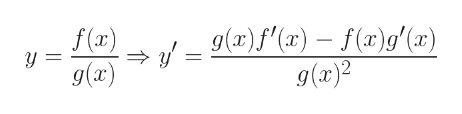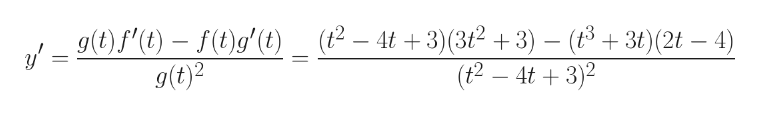2.3 - Can you show the steps to solving this problem?Differentiate.(33) y = (t^3 + 3t)/(t^2 - 4t +3)

Question

2.3 - Can you show the steps to solving this problem?

Differentiate.

(33) y = (t^3 + 3t)/(t^2 - 4t +3)

Step 1

Please see the white board for the quotient rule of differentiation. In this case f(x) = f(t) =  t3 + 3t and g(x) = g(t) = t2 - 4t + 3help_outlineImage Transcriptionclose(x)f"(x) -- f(x)9'(x) g(x)2 f(x) y = g(x) fullscreen
Step 2

Hence, f'(t) = 3t2 + 3 and g'(t) = 2t - 4help_outlineImage Transcriptionclosegf()-()g' (t) _ (2- 4t +3)(32+3)- (83) (2t-4) (t2- 4t3(3t2+3) - (t3t)(2t - 4) (t2 - 4t 3) y g(t)2 _ fullscreen

Want to see the full answer?

See Solution

Want to see this answer and more?

Our solutions are written by experts, many with advanced degrees, and available 24/7

See Solution
Tagged in

Derivative